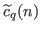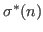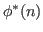## László Tóth: Expansions of arithmetic functions of several variables with respect to certain modified unitary Ramanujan sums, 213-223

### Abstract:

We introduce new analogues of the Ramanujan sums, denoted by, associated with unitary divisors, and obtain results concerning the expansions of arithmetic functions of several variables with respect to the sums. We apply these results to certain functions associated withand, representing the unitary sigma function and unitary phi function, respectively.

Key Words: Ramanujan expansion of arithmetic functions, arithmetic function of several variables, multiplicative function, unitary divisor, sum of unitary divisors, unitary Euler function, unitary Ramanujan sum.

2010 Mathematics Subject Classification: Primary 11A25. Secondary 11N37